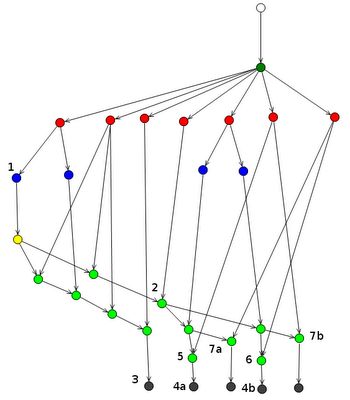## Thursday, November 19, 2009

### Pacman and the importance of BetaNode sharing - Rete Explained

The pacman.drl is starting to shape up, I just added in the additional logic to have pacman slow down during eating. The example is starting to show the value of using a rule engine and hopefully I can use this to explain the interesting characteristics of Rete network and node sharing.

Originally I had just two rules, one that detects when pacman eats normal food and another for when he eats a power pill.
`rule EatFood dialect "mvel" no-loop when  \$char : Character( name == "Pacman" )  \$l : Location( character == \$char )  \$target : Cell( row == \$l.row, col == \$l.col)  \$contents : CellContents( cell == \$target, cellType == CellType.FOOD )  \$s : Score()then  modify( \$contents ) { cellType = CellType.EMPTY };  modify( \$s ) { score += 1 };endrule EatPowerPill dialect "mvel" no-loop when  \$char : Character( name == "Pacman" )  \$l : Location( character == \$char )  \$target : Cell( row == \$l.row, col == \$l.col)  \$contents : CellContents( cell == \$target, cellType == CellType.POWER_PILL )  \$s : Score()then  modify( \$contents ) { cellType = CellType.EMPTY };  modify( \$s ) { score += 5 };end`
Notice those two rules share the first three patterns, but not the forth. This means that the evaluation for that logic only happens once, but works for both rules
I then added a third rule that has monster collision detection. That rule only shares the first pattern. While in the current set of rules only the first pattern is shared here, actually this rule has a larger set of sharing with rules in other packages.
`rule MonsterCollision dialect "mvel" no-loop when  \$pac    : Character( name == "Pacman" )  \$pacLoc : Location( character == \$pac )  \$mon    : Character( name == "Monster" )  \$monLoc : Location( character == \$mon, col == \$pacLoc.col, row == \$pacLoc.row )  \$t : Tick()`
`rule SlowWhenEating dialect "mvel" no-loop when  \$char : Character( name == "Pacman" )  \$l : Location( character == \$char )  \$target : Cell( row == \$l.row, col == \$l.col)  \$contents : CellContents( cell == \$target, cellType == CellType.FOOD || == CellType.POWER_PILL )  \$update : ScheduledLocationUpdate( character == \$char )then  modify ( \$update ) { tock += 2 };end`
This rule adds a few more tocks to the current scheduled location update, effectively adding in a small delay that is perceived as Pacman slowing down. The rule also shares the first three patterns again, with a nice compact syntax for the fourth pattern. But then I thought, hang on the other two rules it shares with, one checks FOOD and the other a POWER_PILL and the logic is also mutually exclusive. If I was to use the 'or' conditional element it would actually generate 2 rules, one for each branch of the logic, and this would allow each to share the fourth pattern. I then changed the rule to this:
`rule SlowWhenEating dialect "mvel" no-loop when  \$char : Character( name == "Pacman" )  \$l : Location( character == \$char )  \$target : Cell( row == \$l.row, col == \$l.col)  (or \$contents : CellContents( cell == \$target, cellType == CellType.FOOD )      \$contents : CellContents( cell == \$target, cellType == CellType.POWER_PILL ) )  \$update : ScheduledLocationUpdate( character == \$char )then  modify ( \$update ) { tock += 2 };end`
If we look at those nodes in the Rete viewer, we get something like below:(click to enlarge)

The first think you'll notice is there are 4 black terminal nodes, yet we have 3 rules. That's because of the 'or', remember an 'or' conditional element actually uses a series of logic transformations to remove the 'or's and instead replacing them with rules that represent each possible outcome - all resulting rules are independent of each other and can match and fire, so be careful as this does not have the same behaviour as an pattern infix '||'.

All four rules share the first pattern:
`\$char : Character( name == "Pacman" )`
The leftmost blue alpha node (1) constraining 'name == "pacman"' is the root node for all rules, so it's tested once and true for all for rules. The connecting yellow node is the left input adapter, which is necessary for the first pattern to allow it to propagate to the green beta nodes.

Terminal 3 is the "MonsterCollision" rule, other than the first shared node, notice that all other patterns, represented by the green beta nodes, which are of the join node type, are independent and exclusive to that rule.

The join node (2) represents the three patterns shared by the "EatFood", "EatPowerPill" and "SlowWhenEating" rules which constrains to the correct Cell:
`Cell( row == \$l.row, col == \$l.col)`
At this point we have a split. 4a and 4b are the two possible outcomes of the "SlowWhenEating" rule, due to the 'or' conditional element. So each shares the 4th pattern, one for FOOD the other for POWER_PILL. Notice that while both outcomes have the pattern the node(5 and 6) is repeated twice :
`\$update : ScheduledLocationUpdate( character == \$char )`
That's because the sharing only happens while the sequences of patterns are the same from the root pattern, once the split occurs the network stays split.

Finally you'll notice 7a and 7b, which refer to the Score pattern of the "EatFood" and "EatPowerPill" rules.

I hope that has given a bit of insight into both Rete and beta node sharing works as well as the current Pacman implementation.